# 12. Graphs

In this chapter, we study two representations of graphs and basic algorithms that use these representations.

Mathematically, a (directed) graph is a pair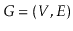where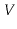is a set of vertices and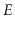is a set of ordered pairs of vertices called edges. An edge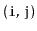is directed from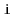to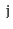;is called the source of the edge andis called the target. A path in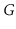is a sequence of vertices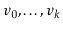such that, for every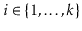, the edgeis in. A pathis a cycle if, additionally, the edge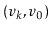is in. A path (or cycle) is simple if all of its vertices are unique. If there is a path from some vertex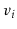to some vertex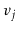then we say thatis reachable from. An example of a graph is shown in Figure 12.1.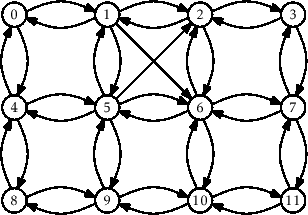Due to their ability to model so many phenomena, graphs have an enormous number of applications. There are many obvious examples. Computer networks can be modelled as graphs, with vertices corresponding to computers and edges corresponding to (directed) communication links between those computers. City streets can be modelled as graphs, with vertices representing intersections and edges representing streets joining consecutive intersections.

Less obvious examples occur as soon as we realize that graphs can model any pairwise relationships within a set. For example, in a university setting we might have a timetable conflict graph whose vertices represent courses offered in the university and in which the edgeis present if and only if there is at least one student that is taking both classand class. Thus, an edge indicates that the exam for classshould not be scheduled at the same time as the exam for class.

Throughout this section, we will use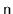to denote the number of vertices ofand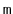to denote the number of edges of. That is,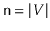and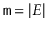. Furthermore, we will assume that. Any other data that we would like to associate with the elements ofcan be stored in an array of length.

Some typical operations performed on graphs are:

•: Add the edgeto.
•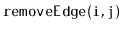: Remove the edgefrom.
•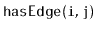: Check if the edge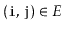•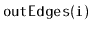: Return a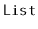of all integerssuch that•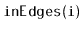: Return aof all integerssuch that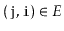Note that these operations are not terribly difficult to implement efficiently. For example, the first three operations can be implemented directly by using a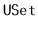, so they can be implemented in constant expected time using the hash tables discussed in Chapter 5. The last two operations can be implemented in constant time by storing, for each vertex, a list of its adjacent vertices.

However, different applications of graphs have different performance requirements for these operations and, ideally, we can use the simplest implementation that satisfies all the application's requirements. For this reason, we discuss two broad categories of graph representations.

Subsections
opendatastructures.org